Function Repository Resource:

# IntegerCompositions

Generate all compositions of an integer into the specified number of parts

Contributed by: Wolfram Staff (original content by Sriram V. Pemmaraju and Steven S. Skiena)
 ResourceFunction["IntegerCompositions"][n,k] gives a list of all compositions of integer n into k parts in canonical order.

## Details and Options

A composition of n in ResourceFunction["IntegerCompositions"] is taken to be a particular arrangement of non-negative integers whose sum is n.

## Examples

### Basic Examples (1)

Get every composition of 5 into three parts:

 In:=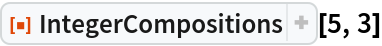Out=### Properties and Relations (6)

The number of compositions of n into k parts is Binomial[n+k-1,n]:

 In:=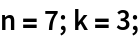In:=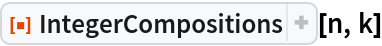Out=In:=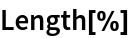Out=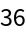In:=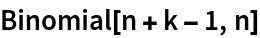Out=All compositions returned by IntegerCompositions are distinct:

 In:=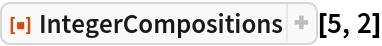Out=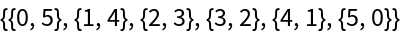In:=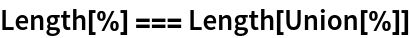Out=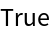Compositions are returned in Sort order:

 In:=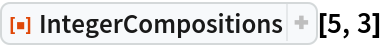Out=In:=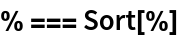Out=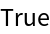Integer compositions are related to integer partitions:

 In:=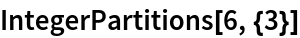Out=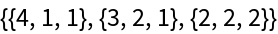In contrast to compositions, partitions do not include 0 and are in non-increasing order:

 In:=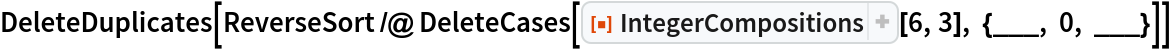Out=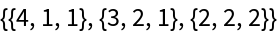If you allow partitions to include 0, then all permutations thereof give the possible compositions:

 In:=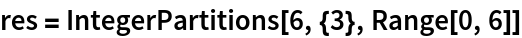Out=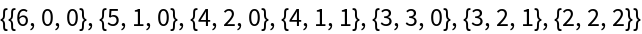In:=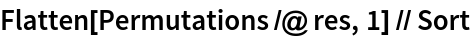Out=In:=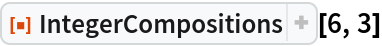Out=While IntegerCompositions allows resulting compositions to include 0, the resource function StrictIntegerCompositions does not:

 In:=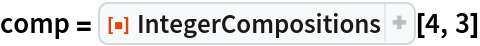Out=In:=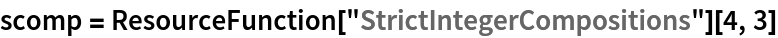Out=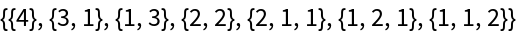In:=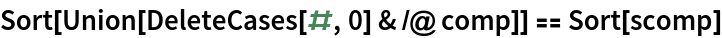Out=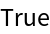## Version History

• 1.0.0 – 06 March 2020